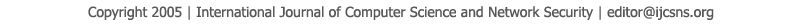To search, Click below search items.All Published Papers Search Service
 Title Author Year Keyword
 Title Bijective Function with Domain in N and Image in the Set of Permutations: An Application to Cryptography Author V?ctor M. Silva-Garc?a, Cornelio Y??ez-M?rquez, Juan L. D?az de Le?n-Santiago Citation Vol. 7  No. 4  pp. 117-124 Abstract In this work an algorithm is constructed that counts n! permutations in n-1 steps. Actually, the algorithm defines a bijective function from the natural numbers to the set of permutations. In addition, for any permutation πL defined over the positions of a string of length L, where L is a multiple of 3, this permutation may be constructed by means of 3 permutations over strings of length ⅔L. This allows for the definition of an iterative cryptosystem over blocks of 96 characters, with numbers in the range of 1090 instead of 10154, approximately. It is also shown that the set of keys grows factorially, so that the number of elements of the set reaches 2500 when working with strings of 96 characters. Finally, by means of an example the iterative cryptosystem using the DES boxes and strings of 96 characters is illustrated. Keywords JV Theorem, Factorial Theorem, Factorial Cryptosystem, Permutations. URL http://paper.ijcsns.org/07_book/200704/20070416.pdf